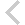FICO Xpress Optimization Examples Repository
 FICO Optimization Community FICO Xpress Optimization HomeFolio - Embedding examples from 'Getting started'

Description
Simple embedding tasks for a portfolio optimization problem:
• executing a Mosel model (folioexec.c)
• parameterized model execution (folioparam.c)
• exporting a matrix (foliomat.c)
• accessing model results (folioobj.c)
• data exchange in memory (runfolio.c,runfoliod.c)
• retrieving solution output from Optimizer callbacks in foliocbio.mos during optimization (runfoliocbio.c)

Source Files

Data Files

foliomemio2.mos

```(!******************************************************
Mosel Example Problems
======================

file foliomemio2.mos
````````````````````
Modeling a MIP problem
to perform portfolio optimization.

Same model as in foliomip3.mos with
simplified data structures (replaced arrays of structured types).
-- Data input/output in memory --
-- Grouping arrays with identical index sets --

Run modes for this model:
1. Stand-alone: data I/O to text files
(run this model from Workbench or from Mosel command line)
2. Submodel to another Mosel model: data exchange in memory
(run model 'runfolio2.mos' to execute this model)
3a. C: I/O to C program folio2.c
(compile and run C program 'folio2.c')
3b. Java: I/O to Java program folio2.java
(compile and run Java program 'folio2.java')
3c. C# (Windows only): I/O to C# program folio2.cs
(compile and run C# program 'folio2.cs')

(c) 2009 Fair Isaac Corporation
author: S.Heipcke, Feb. 2009, rev. Sep. 2018
*******************************************************!)

model "Portfolio optimization with MIP"
uses "mmxprs"

parameters
MAXRISK = 1/3                      ! Max. investment into high-risk values
MINREG = 0.2                       ! Min. investment per geogr. region
MAXREG = 0.5                       ! Max. investment per geogr. region
MAXSEC = 0.25                      ! Max. investment per ind. sector
MAXVAL = 0.2                       ! Max. investment per share
MINVAL = 0.1                       ! Min. investment per share
MAXNUM = 15                        ! Max. number of different assets

DATAFILE = "folio10.dat"           ! File with problem data
OUTPUTFILE = "sol10out.dat"        ! File for solution output
RISKDATA = "RISK"                  ! Locations of input data
RETDATA = "RET"
LOCDATA = "LOCTAB"
SECDATA = "SECTAB"
NUMSHARES = "NUMSHARES"
RETSOL = "RETSOL"
SOLSTATUS = "SOLSTATUS"
end-parameters

declarations
SHARES,S: set of string            ! Set of shares
RISK: set of string                ! Set of high-risk values among shares
REGIONS: set of string             ! Geographical regions
TYPES: set of string               ! Share types (ind. sectors)
LOCTAB: dynamic array(REGIONS,SHARES) of boolean ! Shares per geogr. region
RET: array(SHARES) of real         ! Estimated return in investment
SECTAB: dynamic array(TYPES,SHARES) of boolean ! Shares per industry sector
end-declarations

initializations from DATAFILE
RISK as RISKDATA
RET as RETDATA
LOCTAB as LOCDATA
SECTAB as SECDATA
end-initializations

declarations
frac: array(SHARES) of mpvar      ! Fraction of capital used per share
buy: array(SHARES) of mpvar       ! 1 if asset is in portfolio, 0 otherwise
end-declarations

! Objective: total return
Return:= sum(s in SHARES) RET(s)*frac(s)

! Limit the percentage of high-risk values
sum(s in RISK) frac(s) <= MAXRISK

! Limits on geographical distribution
forall(r in REGIONS) do
sum(s in SHARES | exists(LOCTAB(r,s))) frac(s) >= MINREG
sum(s in SHARES | exists(LOCTAB(r,s))) frac(s) <= MAXREG
end-do

! Diversification across industry sectors
forall(t in TYPES) sum(s in SHARES | exists(SECTAB(t,s))) frac(s) <= MAXSEC

! Spend all the capital
sum(s in SHARES) frac(s) = 1

! Upper bounds on the investment per share
forall(s in SHARES) frac(s) <= MAXVAL

! Limit the total number of assets
sum(s in SHARES) buy(s) <= MAXNUM

forall(s in SHARES) do
buy(s) is_binary                  ! Turn variables into binaries
end-do

! Display Optimizer log
setparam("XPRS_verbose", true)

! Solve the problem
maximize(Return)

! Adapt Mosel comparison tolerance to Optimizer feasibility tolerance
setparam("zerotol", getparam("XPRS_feastol")/10)

! Solution output
function getvalues(v: array(SHARES) of mpvar): dynamic array(S) of real
forall(s in SHARES | v(s).sol<>0) returned(s):= v(s).sol
end-function

initializations to OUTPUTFILE
evaluation of Return.sol as RETSOL
evaluation of sum(s in SHARES | buy(s).sol<>0) 1 as NUMSHARES